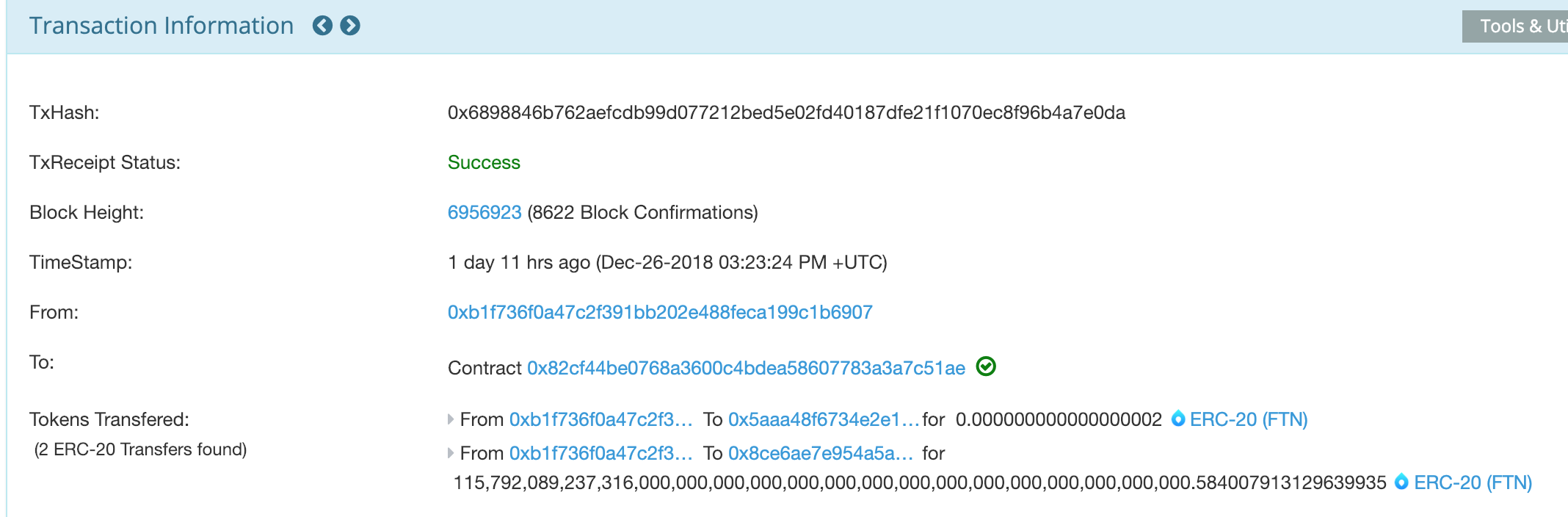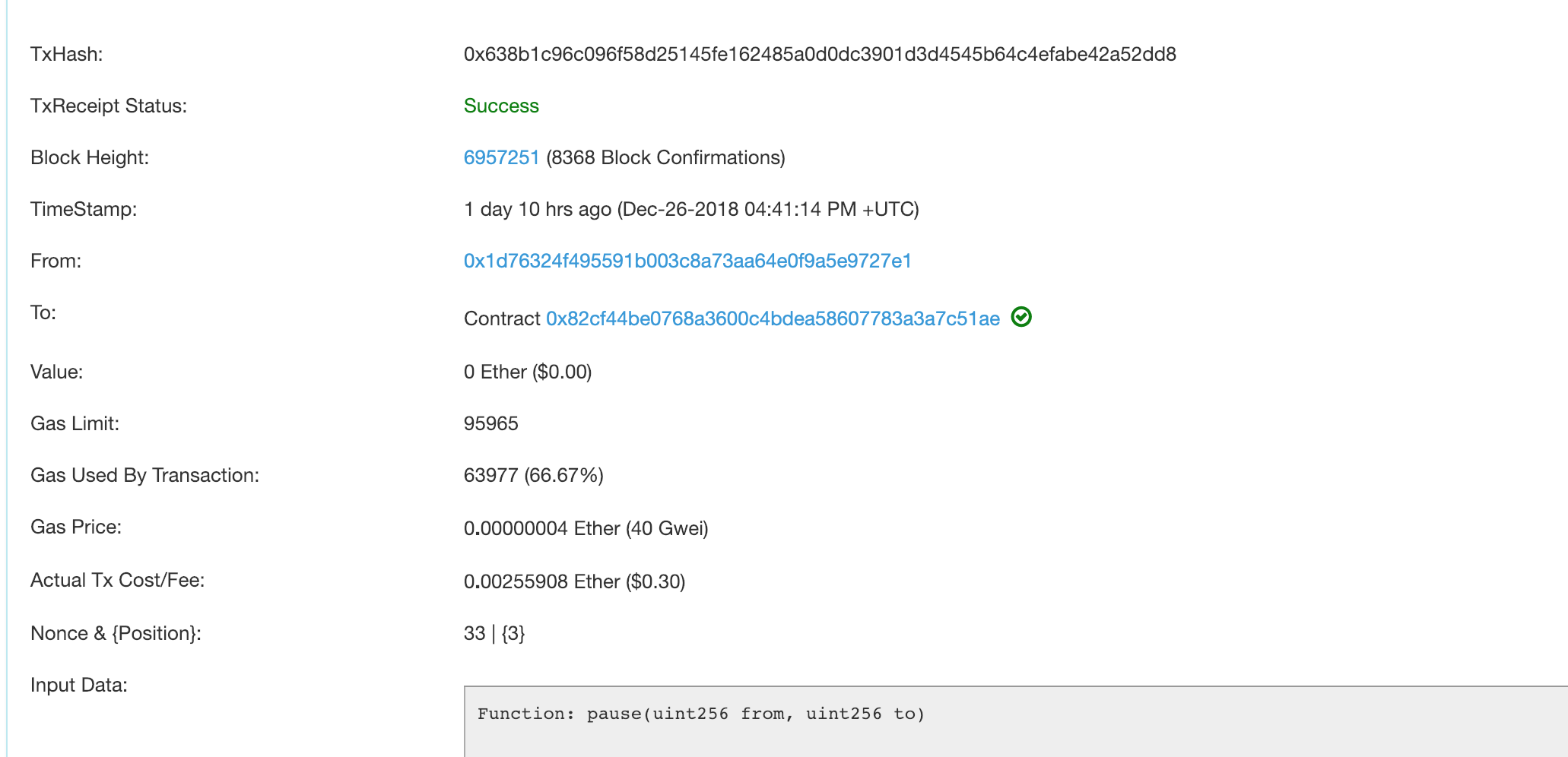FTN 合约漏洞分析

## 0x01 攻击回顾

``````Fountain 项目通证 FTN 于新加坡时间 2018 年 12 月 26 日晚 23 时出现数笔异常链上交易。

``````## 0x02 攻击分析

``````Function: batchTransfers(address[] receivers, uint256[] amounts)

:  0000000000000000000000000000000000000000000000000000000000000040
:  00000000000000000000000000000000000000000000000000000000000000a0
:  0000000000000000000000000000000000000000000000000000000000000002
:  0000000000000000000000005aaa48f6734e2e1c2d7d723fb9182755c9486704
:  0000000000000000000000008ce6ae7e954a5a95ff02161b83308955ebc832cf
:  0000000000000000000000000000000000000000000000000000000000000002
:  0000000000000000000000000000000000000000000000000000000000000002
:  ffffffffffffffffffffffffffffffffffffffffffffffffffffffffffffffff
``````

``````function batchTransfers (address[] receivers, uint256[] amounts) public whenRunning returns (bool) {

uint256 totalAmount = 0;
uint i;
for (i = 0; i < receiveLength; i ++) {
if (r == address(0) || r == owner) continue;
totalAmount += amounts[i];
}
require(totalAmount > 0);
require(canPay(msg.sender, totalAmount));

wallets[msg.sender] -= totalAmount;
uint256 amount;
for (i = 0; i < receiveLength; i++) {
if (r == address(0) || r == owner) continue;
amount = amounts[i];
if (amount == 0) continue;
emit Transfer(msg.sender, r, amount);
}
return true;
}
``````

1. 这里在 for 循环里直接对参数进行累加是合约编写的大忌。因为外部传参是我们无法控制的，非常容易导致数据溢出。
2. 合约攻击往往会从突破 require 语句下手。这次攻击成功，很大可能就是突破了这个代码片段里的两个逻辑上非常合理的 require 语句，使后面的转账操作可以顺利完成。
``````       for (i = 0; i < receiveLength; i ++) {
if (r == address(0) || r == owner) continue;
totalAmount += amounts[i];
}
require(totalAmount > 0);
require(canPay(msg.sender, totalAmount));
``````

## 0x04 忠告

• 发表于 2018-12-28 14:49
• 阅读 ( 783 )
• 学分 ( 5 )
• 分类：安全/漏洞